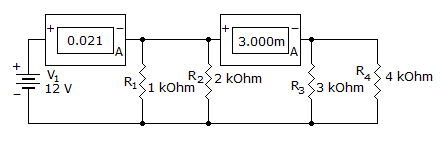# Electronics - Parallel Circuits - Discussion

Discussion Forum : Parallel Circuits - General Questions (Q.No. 25)
25.
Which component is open?R1
R2
R3
R4
Explanation:
No answer description is available. Let's discuss.
Discussion:
17 comments Page 1 of 2.

LAKHAN said:   3 years ago
Thanks for explaining @Mani.

Mani said:   5 years ago
Here we have a total current of the 21m amp,
Current across R1is.
I1=v/R1=12/1*10^3=12m amp

Current across R2 is;
I2=v/R2=12/2*10^3=6m amp,
Remaining current is 3m amp.

Then current across R4 is,
I3=v/R4=12/2*10^3=3m amp,
Hence resistor R3 is open circuit.

Naveen said:   6 years ago
Perfect explanation, Thanks.
(1)

Hima.V.M said:   7 years ago
It's a clear explanation, Thank you @Deepti.

Priya said:   9 years ago
@Kishu is right. We can't connect volt and ammeters directly because their will be short circuit.

Suresh b said:   9 years ago
1 amp = 1000 mA.
0.021 A = 21 mA.

How to calculate total current?

Hi,

Total current = 21mA.

Current required by R1 and R2 = 18mA.

So for remaining 3mA(21mA -18mA), R4 = 4K is enough no need for R3.

Thank you.

Subedar Sk shakya said:   1 decade ago
As we know in parallel circuits current divides& voltage remains same here the first amp meter shows 0.021A is the total current flowing through the R1,R2,R3,R4 and second one shows the current flowing through R3&R4

Now current reading for first one r1*r2/r1+r2=1*2/1+2 =2/3 I=V/R so(12)/(2/3)= 18ma now remains 3ma for second one so it clearly shows again I= V/R =12/4k=3ma So no need of R3.
Here R3 is Open.